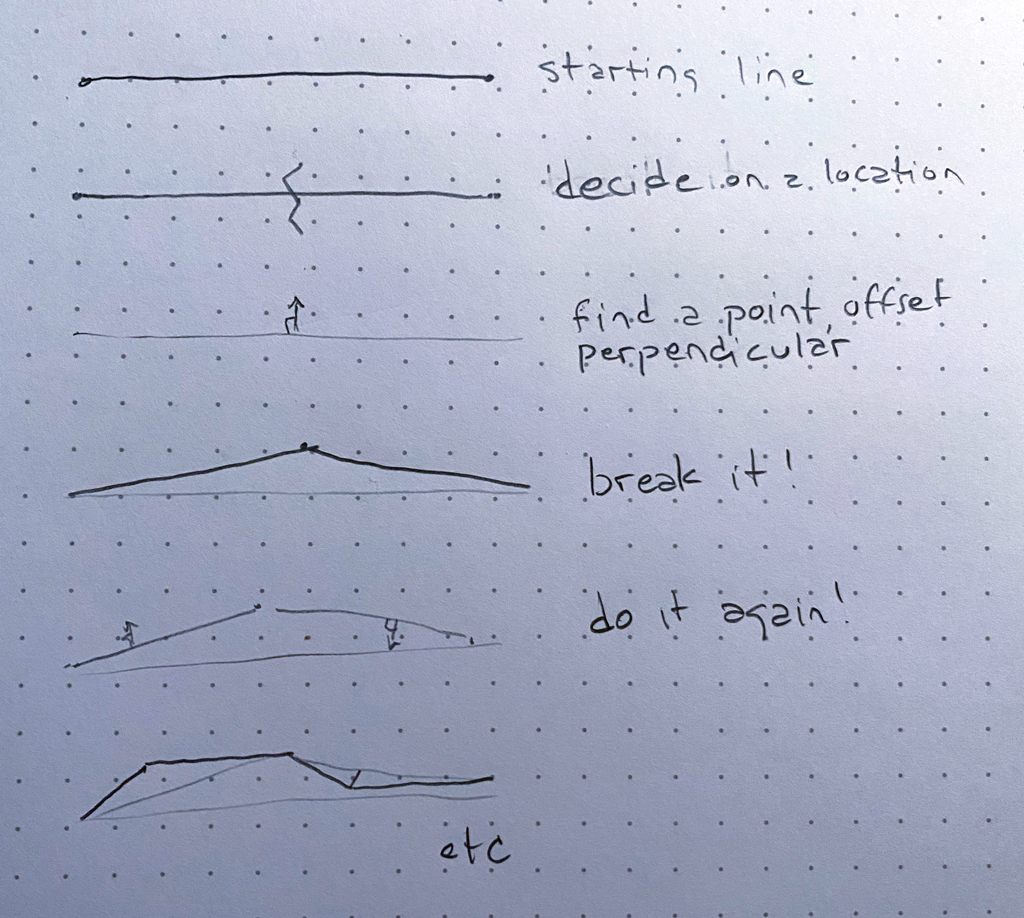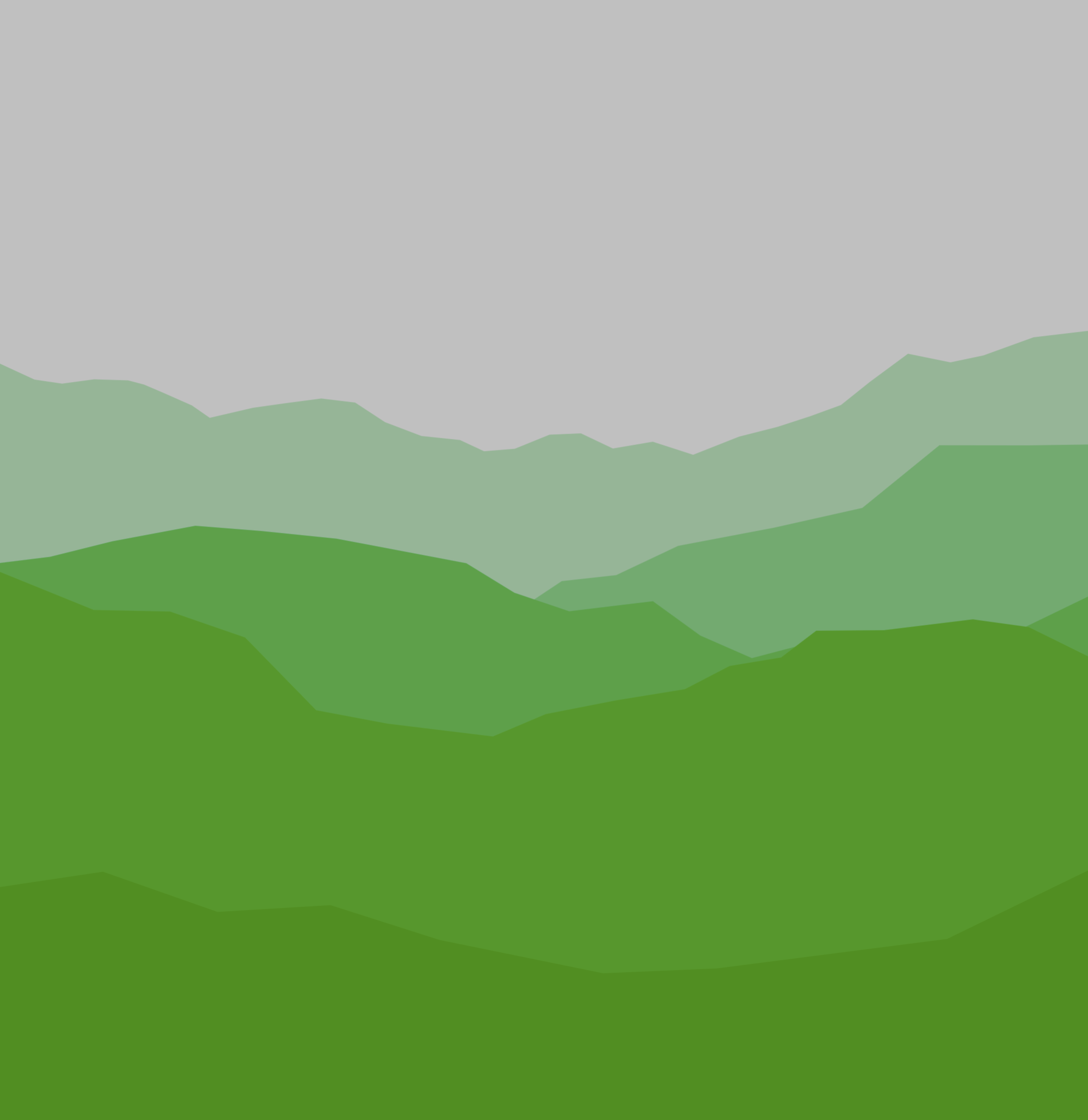Moishe Lettvin

I like coffee, bicycles, camera and code. Currently learning at Recurse Center.

# A Landscape Generator

21 January 2022

One of my favorite things at Recurse so far has been the weekly “Creative Coding” meetup. Every week a group of about half a dozen of us get together and spend about 90 minutes writing code to respond to two randomly selected prompts. For instance, last week one of the prompts was “tech support” so I wrote a thing that creates a Markov chain from the longest Outlook FAQ I could find, and used that chain to build nonsense tech advice. Other people made very clever animations and even interactive games; it’s really amazing what can be done in 90 minutes!

Some people choose to pair and others work independently; I’ve been working independently because working on this kind of problem solo brings me a great deal of joy, but in the spirit of sharing what I’ve learned I want to start writing up some of my code. Be forewarned, this is simple and silly code, but I have learned something new at each of these meetups.

This week the prompts were “hard rain” and “lush green” which to me and others called to mind the Pacific Northwest. I immediately thought of one of my favorite Twitter bots, Soft Landscapes, and wondered if I could make a grey and green version of it.

In the waiting-for-folks-to-arrive pre-meeting time today, we were talking about Turtle graphics, and how you could create fractals with them. So fractals were on my mind.

Many many years ago I remember trying to write some code that would split up a line recursively, offseting the middle of the line a bit, perpendicular to the existing line. Then with the two new lines, doing the same thing again, etc. This is in some ways the ur-fractal. Anyway way back when I tried to do this I gave up on the math of figuring out the perpendicular line to a line for the offset (in my defense I think I was in 7th or 8th grade, and I’ve always been bad at math). Here’s a picture of what I wanted to do:it was that 3rd step that stuck me all those years ago. Now I have some tools available to me that I didn’t have then; yesterday, when I coded this (with the intentional time pressure of the meetup), I chose to use vectors:

``````function perp(l, p, m)
{
let normalized = l.copy().sub(l).setMag(1)
if (Math.random() < 0.5) {
normalized.rotate(-HALF_PI)
} else {
normalized.rotate(HALF_PI)
}
normalized.setMag(m)
}
``````

This function takes three (poorly named!) parameters: `l` is a line specified by two vectors; `p` is the vector along the line where we want to break it, and `m` is the distance we want to move away from the line.

On the first line, we create a vector pointing in the direction of the line with distance 1.

Then we choose whether we’ll break our line “up” or “down” (more correctly clockwise or counterclockwise, but we’re drawing a landscape here), then rotate that vector 90 degrees in the correct direction.

Then, we offset that vector by the point where the line will break. And now we have our new middle vector!

We call that function from a function that fractures the line:

``````function fracture_line(l) {
let offset = Math.random() * 0.2 - 0.1 + 0.5

let midPoint = p5.Vector.lerp(l, l, offset)

midPoint = perp(l, midPoint,
p5.Vector.dist(l, l) / 8.0 * Math.random())

return [l, midPoint, l]
}
``````

That function decides on the middle-ish point, using `lerp`, which will find a point somewhere along the line described by two vectors. I made up some magic numbers which seemed to look good.

After finding the midpoint with the `perp` function, the `fracture_line` function returns an array of three vectors – the original two, and the new offset middle vector.

Then, we can iteratively break the two lines defined by those three vectors, as many times as we’d like:

``````    let current_line = layer_line
for (let i = 0; i < Math.floor(Math.random() * 4) + 3; i++) {
let new_line = []
for (let j = 0; j < current_line.length - 1; j++) {
new_triplet = fracture_line(
[current_line[j], current_line[j + 1]])
new_line = new_line.concat(new_triplet)
}
current_line = Array.from(new_line)
}
``````

In this case we do this between 3 and 6 times, which will leave us with between 8 (2^3) and 64 (2^6) total lines.

Then, we draw a shape whose top is our new collection of lines, and then extends to the bottom of the screen:

``````    let color = p5.Vector.lerp(createVector(192, 192, 192),
createVector(16, 164, 64), (k + 1) / 3)
fill(color.x, color.y, color.z)
beginShape();
for (let i = 0; i < current_line.length; i++) {
vertex(current_line[i].x, current_line[i].y)
}
vertex(width, height)
vertex(0, height)
endShape(CLOSE)
``````

At first I thought about using alpha blending to “layer” the shapes, but realized that didn’t do what I wanted – what I actually wanted was to alpha blend the “fog” over the hills, not the hills over each other and the fog. I simulated this by using our old friend `lerp` to find a point between two vectors, but in this case the vectors were colors! In this case, using RGB-space for the vector worked fine (because I was just blending to gray) but I would like to learn more about blending in other color spaces that match human perception better – a topic for the future!

These calls to fracturing the lines and creating the shapes are wrapped in a loop that decides on 5 starting lines, which kinda-sorta move down the screen:

``````  for (let k = 0; k < 5; k++) {
let layer_line = [
createVector(0, height / 4 + Math.random() * height / 4 + ((k / 3) * height / 4)),
createVector(width, height / 4 + Math.random() * height / 4 + ((k / 3) * height / 4))
]
``````

and that’s really it!

Here’s the source code.

I like the results quite a lot: